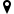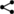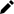Welcome guest
You're not logged in.
284 users online, thereof 0 logged in

## Historical Development of Set Theory

The first strict notion of a set was developed by Bernard Bolzano (1781-1848) who did not use the word “set” but the word “variety”. For him, a “variety” was something, “in which the arrangement of its constituents is unimportant”. In this definition (1847), we can spot a predecessor of today’s extensionality principle – a set is only determined by which elements it has, not by their order, multiplicity, or other properties. Bolzano’s definition implied that sets must have an apparently paradoxical property – a set with infinitely many elements might contain a subset with still contains as many elements as itself.

Richard Dedekind (1831 – 1916) used this weird property as a basis for defining “finite” sets. Moreover, Dedekind was the first mathematician who succeeded in deriving arithmetics and natural numbers from sets. He published these results in his famous work Was sind und was sollen die Zahlen? (translated title: “What are numbers and what do they mean?”) and laid down the basis for the development of the modern set theory.

The actual founder of the modern set theory is Georg Cantor (1845-1918), who made substantial progress in the mathematical concepts related to sets. In particular, he was able to prove that real numbers are uncountable, an unexpected result revealing the fact that there are different types of “infinity”: While the infinitely many natural numbers are countable, there are also infinitely many real numbers, but in some sense “much more” than natural numbers. Cantor made even more groundbreaking discoveries. He also succeeded to show that all points laying on a straight line can be bijectively (i.e. in a 1:1-relationship) mapped to all points of a plane. This discovery forced the mathematicians to re-think the concept of a “dimension”. Cantor also laid down the basis for the idea of ordinal numbers, which provide so-called transfinite methods important for example in algebra or topology.

Cantor used a naive definition for sets we will also start with later in the text of BookofProofs. It makes use of the so-called principle of comprehension which means that whenever we have a property, then we can define a set of elements fulfilling this property. For instance, we can define the set of red cars as $$R:=\{x:x\text{ is a red car}\}.$$ The principle of comprehension seems to be innocuous and good enough to be used for a strict definition of sets. However, if we are careless about the defining property, we might come out with “weird” sets which are not free of paradoxes. Take, for example, the property of “being a set” and define $$S:=\{x:x\text{ is a set}\}.$$ This definition means that $S$ must be self-contained. A theoretical concept, which allows self-contained objects and paradoxes, is not what can be excepted for a foundation of set theory and mathematics and it took not long until other contemporary mathematicians revealed other contradictions. Gottlob Frege (1848-1925) tried in his 1893 published work “Grundgesetze der Arithmetik” (translated title: “Basic Laws of Arithmetics”) to further formalize the set theory of Cantor and to create a foundation of arithmetics from pure logic. In his axiomatic approach, he used the above-mentioned principle of comprehension as one of his axioms. But 1901 Russell showed in his famous Russell’s paradox that Cantor’s naive definition of sets leads to a paradox.

Cantor knew about Russell’s paradox and another one, called the Burali-Forti paradox, but he was not really concerned about them until his death. He was a highly religious man who was convinced that the human mind is simply too limited to understand some infinite sets, and if one cannot conclude that something does not exist, simply if one is not able to understand it. But the vast majority of mathematicians were shocked by the paradoxes and this lead to a thorough revision of the set theory at the beginning of the 20th century.

One approach to revising the set theory was established by Russell himself in collaboration with Alfred Whitehead (1861 – 1947) in their comprehensive work “Principia Mathematica”, and was called the type theory. Basically, it postulated that sets belonged to a hierarchy of types, starting with primary, atomic-type sets, like “numbers” or “points”, which do not contain any other elements. In the type theory, these sets have the $0$-th type. We can then combine elements of $0$-th type to sets of $1$-st type, the sets of $1$-st type to sets of $2$-nd type, etc. This hierarchy forms the set universe. To avoid Russell’s paradox, Russell and Whitehead claimed that the above-mentioned principle of comprehension can be only applied to sets of one type. Comprehensions between different types were forbidden.

Another approach to curing the paradoxes resulting from Cantor’s naive set concept was developed by Ernst Zermelo (1871 – 1953). Unlike Russell and Whitehead, he made do without types for sets and tried to create a more cautious axiomatic system. For instance, he limited the principle of comprehension to the axiom of separation, which postulates that for every property $P$ and every set $X$ there is a subset $$U:=\{x:\text{x is element of }X\text{ and }x\text{ has the property }P\}.$$ We will discuss later how this exactly avoids Russell’s paradox. For now, we will do with the following short explanation: Zermelo allowed only some set-defining properties, he called definite, however, he did not precisely say, what is meant by “definite”. This gap was closed by Albert Skolem (1887 – 1963), who together with Adolf Fraenkel (1891 – 1920) extended Zermelo’s axiomatic system. This work resulted in the currently valid axiomatic system for the set theory, called the Zermelo-Fraenkel-Axioms (ZF).

One of these axioms, the axiom of choice, had been controversially discussed whether it is really independent of the other ZF axioms or can be somehow derived from them. In 1938, Kurt Gödel (1906 – 1978) proved that this assumption is consistent with the remaining ZF axioms. A quarter of a century later, Paul Cohen (1934 – 2007) proved that also its negation is consistent with the remaining ZF axioms. In other words, the axiom of choice is undecidable among the other ZF axioms and it is natural to add it to the axiomatic system ZF. This extended system of axioms is usually abbreviated by ZFC and we use it also in that form in BookofProofs.

Yet another approach to avoid Russell’s paradox was developed by John von Neumann (1903 – 1957), Paul Bernays (1888 – 1977) and Gödel. Unlike Zermelo, they did not limit the principle of comprehension but required that sets build by it can be classified into sets, which can be elements of other sets and those sets, which cannot. Mathematical objects treated in this Neumann-Berneys-Gödel set theory (NBG) are called classes and not sets. Classes, which can be elements of other sets correspond to the usual sets. Classes for which it is forbidden to be elements of other classes are called proper classes. The principle of comprehension reads now as follows: for every property $P$ and every class $X$ there is a subclass $$U:=\{x:\text{x is element of }X\text{ and }x\text{ has the property }P\}.$$ This avoids Russell’s paradox by stating that the “all sets which do not contain themselves” belong to a proper class rather than a set. Neither, there is a self-containing “set of all sets” anymore, since it is simply a “class of all sets”.

Today, ZFA, and NBG systems are considered equivalent approaches to the modern set theory and are often treated equally so in literature. In BookofProofs, we will treat ZFA more in detail.

At this stage, one final note is important. All approaches, the type theory, ZFA, and NBG managed to avoid Russell’s paradox and self-containing sets. However, this does not mean that the set theory based on them is completely free of contradictions (i.e. consistent). Gödel proved in his famous Incompleteness Theorems that we will never know if the set theory is free of contradictions because a proof for its consistency does not exist.|||| created: 2019-01-05 22:49:20 | modified: 2020-08-06 10:10:32 | by: bookofproofs | references: , , , ,